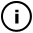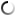# Sunday, October 31, 2010

## VISION (TWO)

//probably there is a better way to code this, but this works for me :)

int first = 0;
int starting =0;
int actual;

int a = 0;
int x = 200;
int y = 400;
int bo;
float GO;
float LEN;
int DUR;
int SIZE;
int hold =150;

void setup()
{
delay(500);
size(480,320);
frameRate(30);
smooth();
noStroke();
background(0);
stroke(255); //colour
fill(255);
}

void draw()
{
fill(0, 10);
rect(0,0,width,height);
if (first==0){intro();}

GO = random(4);
LEN = random(100);
DUR = 1;
SIZE = 6;

bo = 0;

//RIGHT
if (GO<=1){
while (bo<LEN){
delay (DUR);
y++;
fill (255);
if (bo==0){ellipse(y, x, 10, 10);}
rect (y, x, 2 , 2);
bo++;
if (x>=(height-SIZE) || x<=5){x=height/2; y=width/2;}
if (y>=(width-SIZE-SIZE) || y<=5) {x=height/2; y=width/2;}}}

//LEFT
if (GO>1 && GO<2){
while (bo<LEN){
delay (DUR);
y--;
fill (255);
if (bo==0){rect (y, x, 10 , 10);}
rect (y, x, 2 , 2);
bo++;
if (x>=(height-SIZE) || x<=5){x=height/2; y=width/2;}
if (y>=(width-SIZE) || y<=5) {x=height/2; y=width/2;}}}

//DOWN
if (GO>2 && GO<3){
while (bo<LEN){
delay (DUR);
x++;
fill (255);
if (bo==0){ellipse(y, x, 10, 10);}
rect (y, x, 2 , 2);
bo++;
if (x>=(height-SIZE-SIZE) || x<=5){x=height/2; y=width/2;}
if (y>=(width-SIZE) || y<=5) {x=height/2; y=width/2;}}}

if (GO>3 && GO<4){
while (bo<LEN){
delay (DUR);
x--;
fill (255);
if (bo==0){rect (y, x, 10 , 10);}
rect (y, x, 2 , 2);
bo++;
if (x>=(height-SIZE-SIZE) || x<=5){x=height/2; y=width/2;}
if (y>=(width-SIZE) || y<=5) {x=height/2; y=width/2;}}}

}

void intro(){

background(0);
first=1;

}

## infosubmitted by: pixelh8
views: 1436
The Vision (series) is part of a collection of micro apps to explore various aspects of imagery and interactivity. <P> You can find out more information about the similar apps here. <P> http://pixelh8.co.uk/sonusone/ <P><B> All Code & Imagery from this sketch © 2006-2010 Room 1 Studios & Hidden Youth Records UK.</B>

Tags: pixelh8, VISION, (TWO), random

## commentsloading...